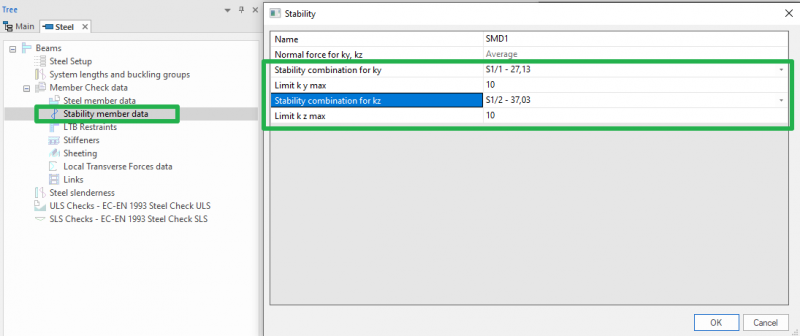# Buckling coefficients

The buckling coefficients ky et kz are, by default, automatically computed by SCIA on the base of two approximated formulas respectively for sway and non-sway structures. These formulas produce buckling coefficients which are, respectively, larger (sway) and smaller (non-sway) than 1.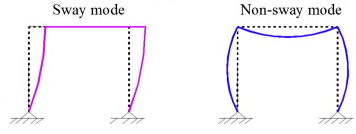The concept of sway or non-sway structure is directly linked to the critical coefficient αcr issue of the stability analysis (see for reference the ECCS 119):

• When αcr ≥ 10, the structure is non-sway, therefore the buckling coefficients will be smaller than 1.
• When αcr < 10, the structure is sway, therefore the buckling coefficients will be larger than 1.

Hence, the user should perform a stability analysis prior to setup the sway-non/sway parameter so to optimize at the best the computed buckling coefficients ky and kz to be used in linear analysis.

• If αcr ≥ 10, then the user can simply perform a linear analysis specifying, in the steel configuration menu, the non-sway behavior of the structure for the y-y and z-z ​​directions, so that the non-sway formula will be used to compute the buckling coefficients of all members.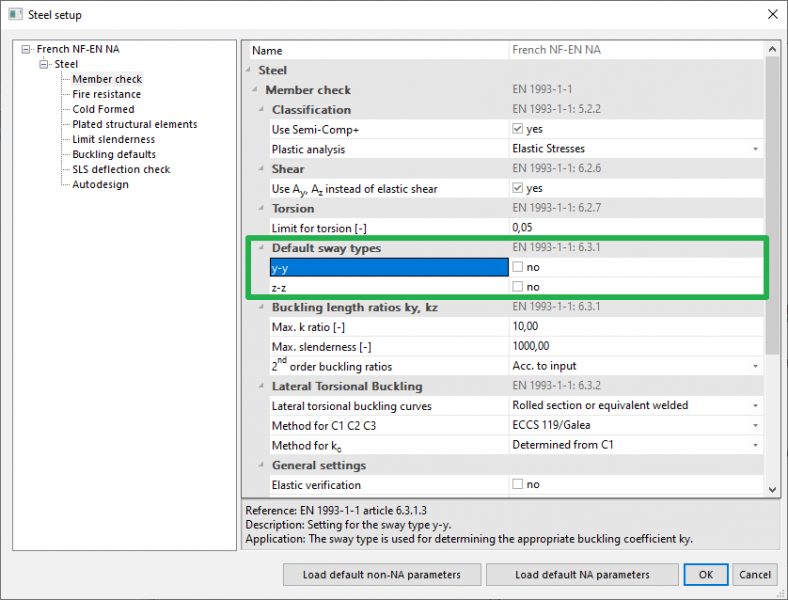• In case αcr <10, the user can choose to perform a linear analysis using sway buckling factors (which are always larger than 1). This method results in a much simpler analysis with respect to a second order analysis accounting for global and local imperfections. However, the user should carefully check that this possibility is allowed in the used design norm (for instance, the Belgian national annex of Eurocode currently does not allow this method, thus requiring a second order analysis). Also, note that this method is more conservative with respect to a second-order calculation accounting for global and local imperfections.

Also important, the formulas used for the computation of ky and kz (available here) are valid only in case of rigid and/or semi-rigid frame structures. This limitation implies that the ky and kz values automatically computed by SCIA should be critically checked when the application case is different from the one specified above.

In such cases several options are available to the user:

• Limitation of the computed ky et kz, to be set in the Steel configuration menu: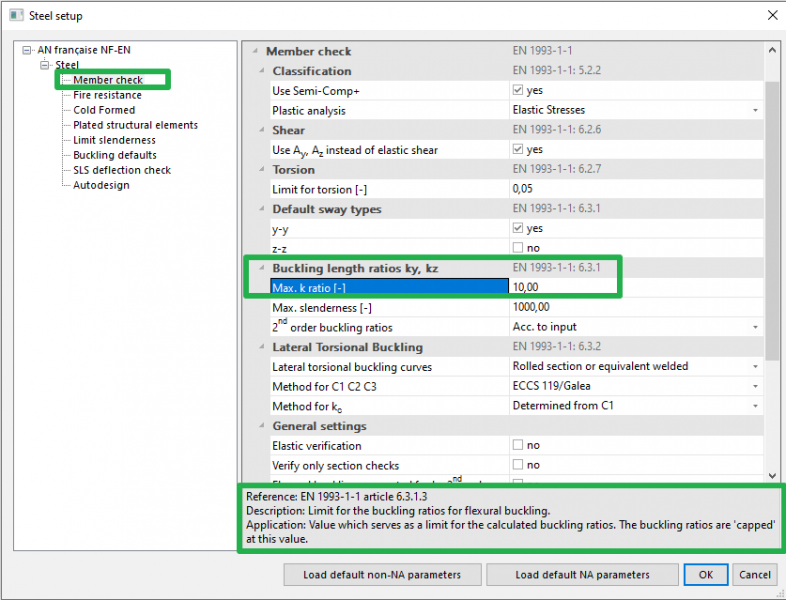• Manual input of the buckling coefficient or buckling length, to be set in the buckling settings: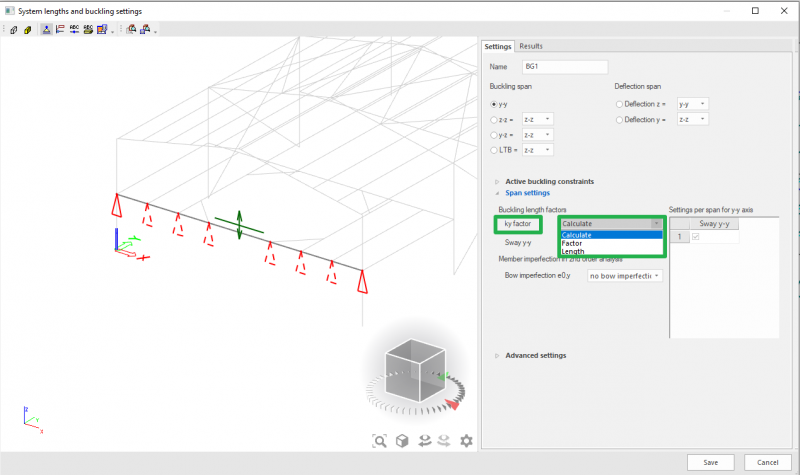• Numerical computation of the buckling coefficient via stability calculation.

In this last case a stability analysis should be performed, and the instability mode of the element for which the ky & kz are looked for should be retrieved. Once this has been done, these stability modes can be affected to the element via the menu steel >member check data>Stability member data: